## Example Questions

### Example Question #1 : How To Find The Square Of Difference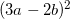can be rewritten as:

Possible Answers: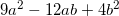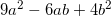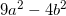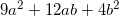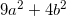Correct answer:Explanation:

Use the formula for solving the square of a difference,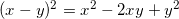. In this case,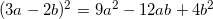### Example Question #2 : How To Find The Square Of Difference

Expand: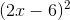Possible Answers: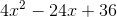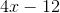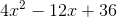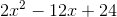Correct answer:Explanation:

To multiply a difference squared, square the first term and add two times the multiplication of the two terms. Then add the second term squared.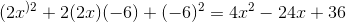### Example Question #1 : How To Find The Square Of Difference

The expressionis equivalent to:

Possible Answers: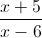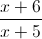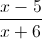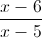Correct answer:Explanation:

First, we need to factor the numerator and denominator separately and cancel out similar terms. We will start with the numerator because it can be factored easily as the difference of two squares.Now factor the quadratic in the denominator.Substitute these factorizations back into the original expression.Theterms cancel out, leaving us with the following answer: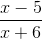### All ACT Math Resources